#### Vol. 24, No. 2, 1968

 Download this articleFor screen For printingRecent Issues Vol. 325: 1 Vol. 324: 1  2 Vol. 323: 1  2 Vol. 322: 1  2 Vol. 321: 1  2 Vol. 320: 1  2 Vol. 319: 1  2 Vol. 318: 1  2Online Archive Volume: Issue:The Journal Subscriptions Editorial Board Officers Contacts Submission Guidelines Submission Form Policies for Authors ISSN: 1945-5844 (e-only) ISSN: 0030-8730 (print) Special Issues Author Index To Appear Other MSP Journals
Functions with real poles and zeros

### Raymond Moos Redheffer

Vol. 24 (1968), No. 2, 345–378
##### Abstract

Throughout this paper {λn} is a real sequence with λn0 and λn λn+1,−∞ < n < . The counting function Λ1(u) is the number of λn between 0 and u, counted negatively for negative u. Similarly μn is a real sequence with μn0 and with counting function Λ2(u). In this summary (which corresponds to the case p = 1 of the paper) we define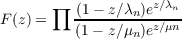by taking all factors for which λn and ∕xn lie on the interval (R,R) and then letting R →∞. Our objective is to obtain conditions on the growth of F(z) from conditions on the function Λ(u) = Λ1(u) Λ2(u).

Denoting the even part of Λ(u) by Λe(u), we can state our first result as follows: Suppose Λ(u) = O(u) and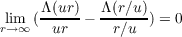for each u0. Then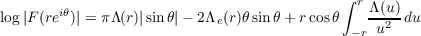apart from an error term ηr log |2csc𝜃|, where η 0 uniformly in 0 < |𝜃| < π as r →∞. This improves theorems of Pfluger, Kahane and Rubel, Cartwright, and others, in that we do not assume existence of limΛ(u)∕u, we do not assume that F is entire or even, and the error term has a convergent integral with respect to 𝜃. Similar theorems for functions with negative poles and zeros, given later in the paper, generalize other familiar results. Here the error term involves log(2sec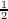𝜃).

Another kind of result is briefly described as follows: Let R = R(x) and S = S(x) be positive functions such that the ratios x∕R,R∕x,x∕S,S∕x are bounded as |x|→∞. Then for many purposes the function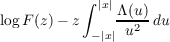can be replaced by the function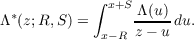This remark is given content by detailed estimates of the error and of Λ. (For simplicity of statement the text takes R = S but the form mentioned here is sometimes more convenient, and is allowed by the proofs.) The development leads to a simple, systematic method of proving variety of growth theorems. Formulation of such a method is a principal goal of this paper, and our specific examples are intended only for illustration.

Primary: 30.60
##### Milestones